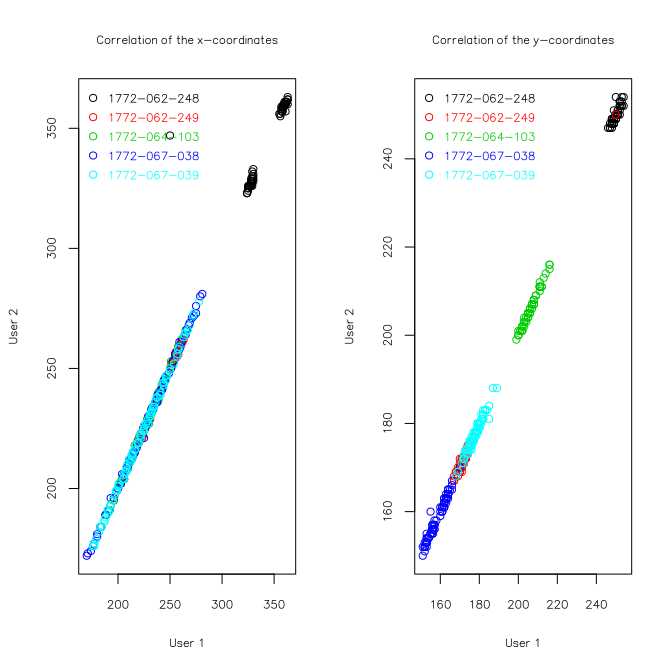``````## Error in eval(expr, envir, enclos): object 'opts_chunk' not found
``````
``````scriptName <- "SetArea_Measure_UserConfirm.ijm"
pdfName <- "Usage_of_ImageJ_macro_SetArea_Measure_UserConfirm.pdf"
resultsUser1 <- "Results_SetArea_Measure_UserConfirm_User1.txt"
resultsUser2 <- "Results_SetArea_Measure_UserConfirm_User2.txt"
``````

## Summary

Images of Fucci cells loaded in the C1 capture array, were taken before cell lysis. Three modes have been used for imaging: bright field (BF), Green and Red. Raw images in the Cellomics specific C01 format were used for measuring fluorescence intensities and reporting errors in Fiji ImageJ. All platforms version by using the macro SetArea_Measure_UserConfirm.ijm. The usage of this macro is illustrated in the accompanying PDF file Usage_of_ImageJ_macro_SetArea_Measure_UserConfirm.pdf. The macro will ask the user to select the directory which contains all the raw image files. The following procedures are done:

• Open images one by one: first bright field, then Green, then Red channel images
• Draw a circular area with a diameter of 13 pixels on the bright field image
• Open dialogue box and and wait for the user to place the circle over the cell
• Measure fluorescence intensities on this defined area for teh Green and Red channel image, and measure background intensity levels 100 x 50 pixels adjacent to the cell (unless position is changed by the user)
• Open dialogue box and ask the user for an error report: comment and error type
• Record the file name, coordinates, size of area, mean intnsity, standard deviation, minimum and maximum intensities and an Error report
• Repeat the previous steps for every set of three images in the folder

The Fiji ImageJ macro was run independently by Elo Madissoon, User 1 (file Results_SetArea_Measure_UserConfirm_User1.txt) and Michael Böttcher, User 2 (file Results_SetArea_Measure_UserConfirm_User2.txt). The current document explains the column names in the results file, analyzes the consistency of the measurements, compares and finds consensus in the error reporting and gives an overview of the final values.

### `cell_id`

Identifier of the well, which is the common part of the filename for all channels (bright field, red and green)

### `cell_coord_x` and `cell_coord_y`

Pixel coordinates of the upper left corner of the smallest rectangle that completely contain the selection area.

### `area`

Size of the selection area.

### `mean`, `std`, `min`, `max`

mean, standard deviation, minimum and maximum fluorescence intensity in the selection. Corresponding measurements to the background area (adjacent to the cell) when prefix is “bg.” and to green channel or red channel when the suffix is “.ch2” or “.ch3” correspondingly.

### `Error`

The error types:

• “0-No Error” (default)
• “1-No cell”
• “2-Debris”
• “3-OutOfFocus”
• “4-MultipleCells”

### `Comment`

Write additional notes here, if necessary. Deafults to “No comment”

### `Fluo_QC`

A boolean value is given to each cell depending on the error type (e.g. “0-No Error” corresponds to FALSE)

### Consistency of ImageJ measurements

Load the fluorescence results files and summarise the content.

``````panel.cor <- function(x, y, digits=2, prefix="", cex.cor) {
usr <- par("usr"); on.exit(par(usr))
par(usr = c(0, 1, 0, 1))
r <- abs(cor(x, y))
txt <- format(c(r, 0.123456789), digits=digits)
txt <- paste(prefix, txt, sep="")
if(missing(cex.cor)) cex <- 0.8/strwidth(txt)

test <- cor.test(x,y)

signif <- symnum(test\$p.value, corr = FALSE, na = FALSE,
cutpoints = c(0, 0.001, 0.01, 0.05, 0.1, 1),
symbols = c("***", "**", "*", ".", " "))

text(0.5, 0.5, txt, cex = cex * r)
text(.8, .8, signif, cex=cex, col=2)
}

colnames(user2)
``````
``````##   "cell_id"        "cell_coord_x"   "cell_coord_y"   "area"           "mean_ch2"       "std_ch2"
##   "min_ch2"        "max_ch2"        "bg_coord_ch2_x" "bg_coord_ch2_y" "bg_area_ch2"    "bg_mean_ch2"
##  "bg_std_ch2"     "bg_min_ch2"     "bg_max_ch2"     "area_ch3"       "mean_ch3"       "std_ch3"
##  "min_ch3"        "max_ch3"        "bg_coord_ch3_x" "bg_coord_ch3_y" "bg_area_ch3"    "bg_mean_ch3"
##  "bg_std_ch3"     "bg_min_ch3"     "bg_max_ch3"     "Error"          "Comment"
``````

The cell location has to be manually set for all bright field images. The correalation of the cell coordinates reflects reproducibility of the cell location determination. The correlation of fluorescence intensities reflects the reproducibility of the results. The correlation between cell coordinates and fluorescence values is plotted, color coded by Run.

``````subb <- substr(user1\$cell_id, 1, 12)
mfrow.orig <- par(mfrow=c(1,2))
plot(user1\$cell_coord_x, user2\$cell_coord_x, col=as.factor(subb), main="Correlation of the x-coordinates", xlab="User 1", ylab="User 2")
legend("topleft", legend=c(levels(as.factor(subb))), pch=1, col=c(1:5), text.col=c(1:5), bty="n")
plot(user1\$cell_coord_y, user2\$cell_coord_y, col=as.factor(subb), main="Correlation of the y-coordinates", xlab="User 1", ylab="User 2")
legend("topleft", legend=c(levels(as.factor(subb))), pch=1, col=c(1:5), text.col=c(1:5), bty="n")
````````````plot(user1\$mean_ch2, user2\$mean_ch2, main="Correlation of measures in Ch2, green", xlab="User 1", ylab="User 2")
legend("topleft", legend=c(levels(as.factor(subb))), pch=1, col=c(1:5), text.col=c(1:5), bty="n")
plot(user1\$mean_ch3, user2\$mean_ch3, main="Correlation of measures in Ch3, red", xlab="User 1", ylab="User 2")
legend("topleft", legend=c(levels(as.factor(subb))), pch=1, col=c(1:5), text.col=c(1:5), bty="n")
``````